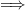# Difference between revisions of "Module Runoff"

Jump to: navigation, search

## Overview

Module Runoff allows the calculation of the overland surface runoff over a grid as function of the water column slopes between adjacen cells (dynamic wave). The water column, namely the water located above the terrain, is given by the Module Basin after considering the precipitation input and the losses due to the evaporation and the infiltration.

## Main Processes

The ModuleRunoff computes flow at cell faces using three different approaches (user option)

1. Full St. Venant Equation in 2D (shallow water equations)
2. Manning Equation with surface water gradient (diffuse wave)
3. Manning Equation with bottom gradient (kinematic wave)

### St. Venant Equations

The St. Venant Equations are derived from the Newton's second law taking into account inertia effects. In this case a 2D approach is followed. See equations description See equations description.

### Manning Equation

The overland surface runoff flow (m3/s) is calculated at the cell faces and it can be obtained by applying the Manning's equation. In this case inertia effects are neglected and forces balance bottom friction.

<ref>Gauckler, P. (1867), Etudes Théoriques et Pratiques sur l'Ecoulement et le Mouvement des Eaux, Comptes Rendues de l'Académie des Sciences, Paris, France, Tome 64, pp. 818–822</ref> :

$1}{n}\cdot A\cdot R_{h}^{2/3}\cdot s_i^{1/2}\hspace {5.7cm$

where:

 Qi is the overland flow in each direction (m3/s) A is the area of the cross-section (m2) n is the Manning coefficient (s/m1/3) Rh is the hydraulic radius (m) Si is the slope of the water surface (m/m)

#### Hydraulic radius

In rectangular channels, the hydraulic radius is evaluated by the formula:

$h}=\frac{w\cdot h}{w+2\cdot h}\hspace {6.8cm$

But in runoff the lateral cell face is open boundary (to the next cell) not exerting fricition in a surface as the bottom boundary, and so hydraulic radius can be rewritten:

$h}=\frac{w\cdot h}{w}\hspace {6.8cm$

and the hydraulic radius is Rh=h. Therefore the Manning's equation can be rewritten as:

$1}{n}\cdot w\cdot h^{5/3}\cdot s^{1/2}\hspace {5.8cm$

#### Slope

The slope (s) is calculated by the difference of the water levels (H) at the extremities of the considered cell:

$5.5cm$

where:

 H is the water level (m) h(i,j) is the water column (m) T(i,j) is the Topography (m) j is X direction i is Y direction

$x}=\frac{H(i,j-1)-H(i,j)}{DZX}\hspace {5.1cm$

where:

 sx is the slope in the X direction (m) H(i,j-1) is the water column at the left face of the cell (m) H(i,j) is the water column at the right face of the cell (m) DZX is width of the cell in the X direction (m)

$y}=\frac{H(i-1,j)-H(i,j)}{DZY}\hspace {5.1cm$

where:

 sy is the slope in the Y direction (m) H(i-1,j) is the water column at the left face of the cell (m) H(i,j) is the water column at the right face of the cell (m) DZY is width of the cell in the X direction (m)

In order to take in account the limitation given by the Manning's equation (1.1) that tends to overestimate the flow velocity when solpe > 0.04, the slope value obtained by the formulas (1.5) and (1.6) it is subsequently adjusted by the following function: Slope correction given by City of Albuquerque, 1997, p.22-26

$(-62.38\cdot s)}\hspace {2.4cm$

where:

 s is the slope (m)

### Manning cofficient

The Manning coefficient is derived from the land use map. Indeed by using a GIS program it is possible to associate at each cell a land use class in order to obtain, by the support of an abacus or table, a Manning coefficent value. See the Other Features section.

### Connection with River

In the eventuality presence of a river it is possible to obtain two different configurations:

• Flow to the river when the water level of the river is lower that soil one

• Flow from the river when the water level is higher than the soil one

The flow between river and runoff is computed using the same formulation as in runoff cells using the surface gradient between runoff and river.

## Boundary Conditions

In Runoff there is the option to define the boundary condition as a level. This level will be imposed at the boundary and the water will exit if the water level is higher than the boudary level.

### Computation

The boundary fluxes are computed after the flow computation iteration. The flux may occur if the runoff level in boundary cells is higher than imposed level. Boundary flux is computed with celerity

Q = cel * A


where cel is celerity where A is vertical Area for flux

### Keywords

The keyword in Runoff_X.dat that connects the open boundary is:

IMPOSE_BOUNDARY_VALUE     : 1


The keyword that defines the boundary level is:

BOUNDARY_VALUE            : 100.


The open boundary computation can be limited to specific areas defining the maximum altimetry that the boundary will be open. This is specifically useful when one wants to open the surface water at flat areas where in fact the flux can go trough the boundaries of the watershed delimitation.

MAX_DTM_FOR_BOUNDARY      : 1000.


Using a value of the latter keyword higher than the maximum altimetry found in the watershed will make the boundary open in all watershed.

## Other Features

### How To Generate Manning Coefficients

Manning coefficients must be provided in runoff data file.

Options:

• Use a constant value
• Define a Manning's grid: One possible option is to associate Manning with land use classes (shape file). In this case can use MOHID GIS going to menu [Tools]$\Longrightarrow$ [Shape to Grid Data] and provide:
 (i) the grid (model grid) ii) the land use shape file iii) the corespondence between land use codes and Manning

Use Manning inicialization with Module FillMatrix standards in the block:

<BeginOverLandCoefficient>
FILE_IN_TIME           : NONE
INITIALIZATION_METHOD  : ASCII_FILE
REMAIN_CONSTANT        : 1
DEFAULTVALUE           : 0.08
FILENAME               : ..\..\GeneralData\Runoff\Mannings200m_v2.dat
<EndOverLandCoefficient>